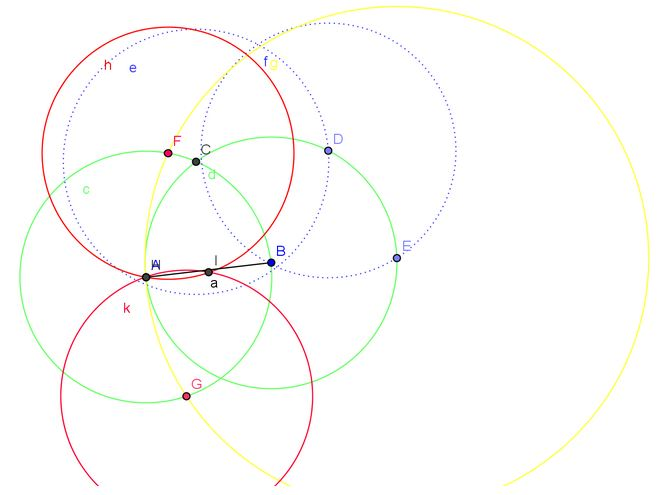## 仅用圆规找中点1. 分别以 $$A,B$$ 为圆心, $$AB$$ 为半径画圆(绿色)交于 $$C$$;
2. 在圆 $$B$$ 上取 $$D,E$$ 两点, 使 $$CD=DE=AB$$, 则 $$AE=2AB$$;
3. 以 $$E$$ 为圆心,  $$AE$$ 为半径画圆(黄色)交圆 $$A$$ 于  $$F,G$$;
4. 分別以 $$F,G$$ 为圆心, $$AF$$ 为半径画圆(红色), 交于 $$A,I$$ 两点, 则 $$I$$ 就是 $$AB$$ 中点.

This site uses Akismet to reduce spam. Learn how your comment data is processed.